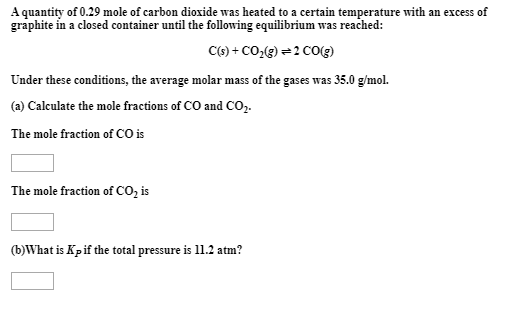# A quantity of 0.29 mole of carbon dioxide was heated to a certain temperature with an excess of graphite in a closed container until the following equilibrium was reached: C(s) + CO2(g) ⇌ 2 CO(g) Under these conditions, the average molar mass of the gas was 35.0 g/mol. (a) Calculate the mole fractions of the CO and CO2. (b) What is the Kp if the total pressure is 11.2 atm?